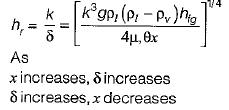Courses

# Condensation And Boiling

## 10 Questions MCQ Test | Condensation And Boiling

Description
This mock test of Condensation And Boiling for Mechanical Engineering helps you for every Mechanical Engineering entrance exam. This contains 10 Multiple Choice Questions for Mechanical Engineering Condensation And Boiling (mcq) to study with solutions a complete question bank. The solved questions answers in this Condensation And Boiling quiz give you a good mix of easy questions and tough questions. Mechanical Engineering students definitely take this Condensation And Boiling exercise for a better result in the exam. You can find other Condensation And Boiling extra questions, long questions & short questions for Mechanical Engineering on EduRev as well by searching above.
QUESTION: 1

### Nusselt number in Boiling and Condensation process is prescribed by the function

Solution:

For condensation or boiling, the convection coefficient depends upon ΔT = (Tw- Tsat), the body force arising from the liquid-vapour density difference, g ( ρi - pv) , the latent heat hfg, the surface tension σ, a characteristic length L and the thermophysical properties of the liquid or vapour p, cp, μ, and K. From dimensionless analysis,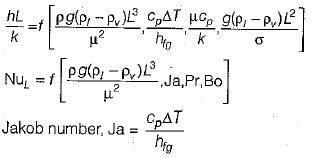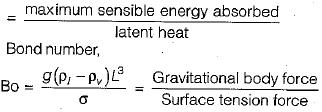QUESTION: 2

Solution:
QUESTION: 3

### All of the following assumptions for Nusselt’s theory of laminar film condensation on a vertical plate is correct except

Solution: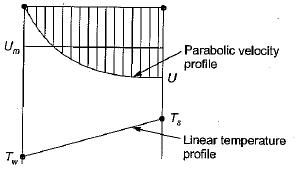QUESTION: 4

Consider boiling curve for pool boiling of water at saturation temperature and atmospheric pressure and match the following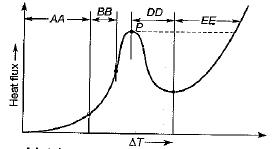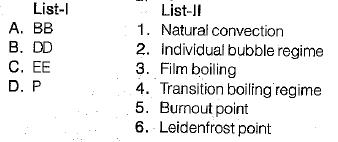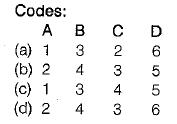Solution: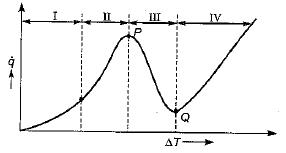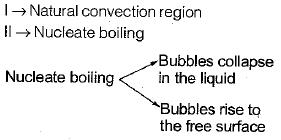III Transition boiling
IV Film boiling Point

P→ Maximum / Critical heat flux or Burnout point
Point Q → Minimum heat flux (Leidenfrost point) Point Pis also known as boiling crisis indicating the onset of departure from nucleate boiling.

QUESTION: 5

The convective coefficients for boiling and condensation usually lie in the range (W/m2-K)

Solution:
QUESTION: 6

Dropwise condensation usually occurs on

Solution:
QUESTION: 7

Consider the following statements:
1. If a condensing liquid does not wet a surface, then dropwise condensation will not take place un it:
2. Dropwise condensation gives a higher transfer rate than filmwise condensation.
3. Reynolds number of condensing liquid is based on its mass flow.
4. Suitable coating or vapour additive is used to promote filmwise condensation.

Which of these statements are correct?

Solution:
QUESTION: 8

5 In condensation over a vertical surface, the value of convection coefficient varies as

Where k is the thermal conductivity of the liquid

Solution:

The average heat transfer coefficient is given by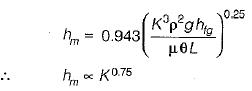QUESTION: 9

Which of the followings is a wrong statement in the context of convective heat transfer coefficient in laminar film condensation?The heat transfer coefficient varies as

Solution: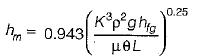From this equation: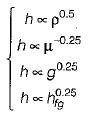QUESTION: 10

For filmwise condensation on a vertical plane; the film thickness 5 and heat transfer coefficient h vary with distance* from the leading edge as

Solution: